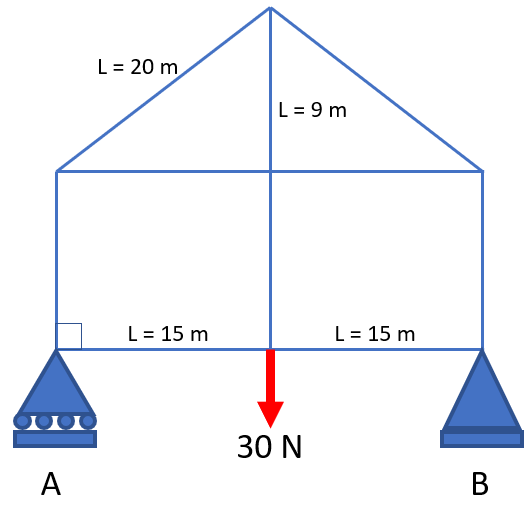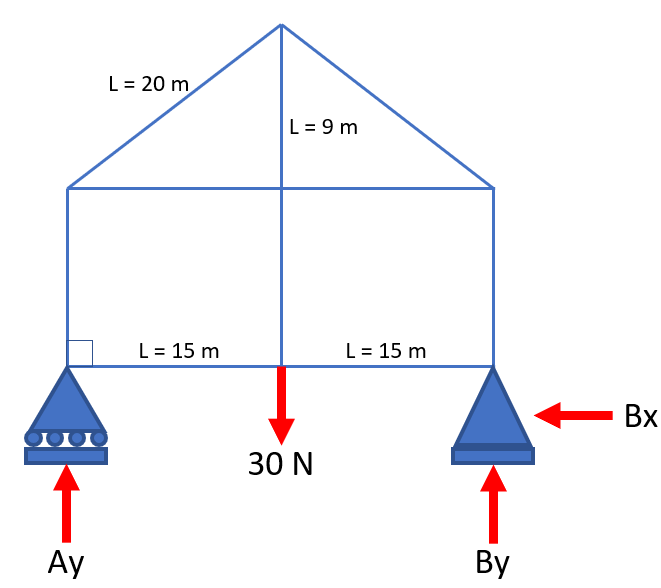## Truss

In the truss diagram below, calculate the reaction at point A.Hint
The problem is asking to solve for $$A_y$$ in the free body diagram below:Hint 2
Due to point A being a roller support, there is only a vertical component force $$A_y$$ . Point B is a fixed support, so there is both a vertical and horizontal component ( $$B_y$$ and $$B_x$$ respectively). The problem statement is asking for the reaction at point A, so let's analyze the moments about point B.
The problem is asking to solve for $$A_y$$ in the free body diagram below:Due to point A being a roller support, there is only a vertical component force $$A_y$$ . Point B is a fixed support, so there is both a vertical and horizontal component ( $$B_y$$ and $$B_x$$ respectively). The problem statement is asking for the reaction at point A, so let's analyze the moments about point B.

Assuming clockwise is positive, the sum of moment about B are zero:
$$\sum M_B=0$$$Remember, Moment = Force Applied at Point x Distance to Point $$\sum M_B=0=30N(15m)-A_y(15m+15m)$$$
$$A_y(30m)=450N\cdot m$$$$$A_y=\frac{450N\cdot m}{30m}=15\:N$$$
15 N